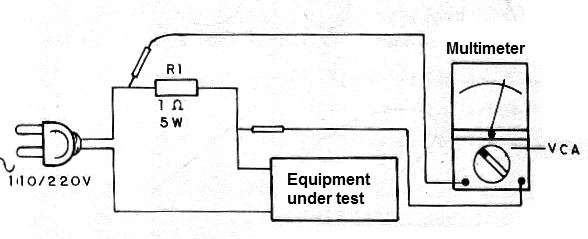### DatasheetsN° of component

## Measurement of the Power of an AC Circuit (Wattmeter) (INS041E)

With the procedure we describe, we can measure the power consumed by devices connected to the power grid for values ??up to 500 W in the 110 V network and 1000 W in the 220 V network. Powers less than 40 W, however will not be measured with precision given the non-linearity of the diodes in the current low range. In the case of electronic or digital multimeters this problem does not exist. The instruments and material required are:

a) Voltmeter or multimeter for alternating voltages

b) R1 - 1 ohm resistor x 5 W

Procedure

The figure below shows how to connect the elements to the measurements.What is done is to measure the alternating voltage on a resistor of known value. Being too low, it does not affect the power of the device. Simply multiply the value in volts measured by the power grid voltage to obtain the consumption. For example, 1 Volt over 1 ohm means 1 A and in the 110 V network this represents 110 W. 1 volt over 1 ohm in 220 V represents 2.0 A and this means 220 W. We proceed as follows:

We put the multimeter on the lowest alternating voltage scale

We connect it to the resistor as indicated

We turn on the device under test

We multiply the voltage measured by the power grid voltage to have the power consumed.